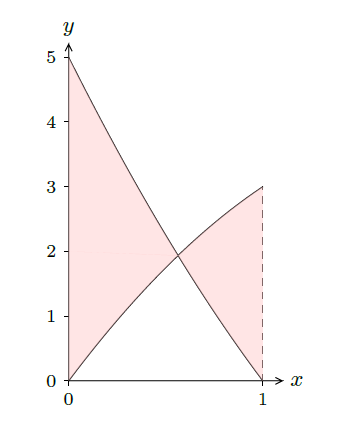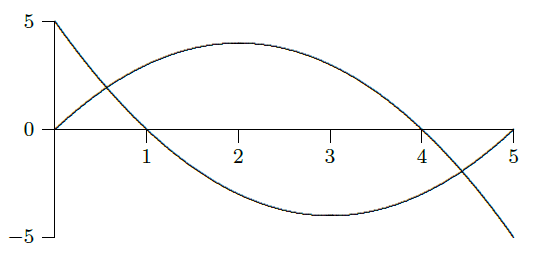$$\newcommand{\id}{\mathrm{id}}$$ $$\newcommand{\Span}{\mathrm{span}}$$ $$\newcommand{\kernel}{\mathrm{null}\,}$$ $$\newcommand{\range}{\mathrm{range}\,}$$ $$\newcommand{\RealPart}{\mathrm{Re}}$$ $$\newcommand{\ImaginaryPart}{\mathrm{Im}}$$ $$\newcommand{\Argument}{\mathrm{Arg}}$$ $$\newcommand{\norm}{\| #1 \|}$$ $$\newcommand{\inner}{\langle #1, #2 \rangle}$$ $$\newcommand{\Span}{\mathrm{span}}$$

# 9.1: Area Between Curves

•• Contributed by David Guichard
• Professor (Mathematics) at Whitman College
$$\newcommand{\vecs}{\overset { \rightharpoonup} {\mathbf{#1}} }$$ $$\newcommand{\vecd}{\overset{-\!-\!\rightharpoonup}{\vphantom{a}\smash {#1}}}$$$$\newcommand{\id}{\mathrm{id}}$$ $$\newcommand{\Span}{\mathrm{span}}$$ $$\newcommand{\kernel}{\mathrm{null}\,}$$ $$\newcommand{\range}{\mathrm{range}\,}$$ $$\newcommand{\RealPart}{\mathrm{Re}}$$ $$\newcommand{\ImaginaryPart}{\mathrm{Im}}$$ $$\newcommand{\Argument}{\mathrm{Arg}}$$ $$\newcommand{\norm}{\| #1 \|}$$ $$\newcommand{\inner}{\langle #1, #2 \rangle}$$ $$\newcommand{\Span}{\mathrm{span}}$$ $$\newcommand{\id}{\mathrm{id}}$$ $$\newcommand{\Span}{\mathrm{span}}$$ $$\newcommand{\kernel}{\mathrm{null}\,}$$ $$\newcommand{\range}{\mathrm{range}\,}$$ $$\newcommand{\RealPart}{\mathrm{Re}}$$ $$\newcommand{\ImaginaryPart}{\mathrm{Im}}$$ $$\newcommand{\Argument}{\mathrm{Arg}}$$ $$\newcommand{\norm}{\| #1 \|}$$ $$\newcommand{\inner}{\langle #1, #2 \rangle}$$ $$\newcommand{\Span}{\mathrm{span}}$$

We have seen how integration can be used to find an area between a curve and the $$x$$-axis. With very little change we can find some areas between curves; indeed, the area between a curve and the $$x$$-axis may be interpreted as the area between the curve and a second "curve'' with equation $$y=0$$. In the simplest of cases, the idea is quite easy to understand.

Example 9.1.1

Find the area below $$f(x)= -x^2+4x+3$$ and above $$g(x)=-x^3+7x^2-10x+5$$ over the interval $$1\le x\le2$$.

Solution

In figure 9.1.1 we show the two curves together, with the desired area shaded, then $$f$$ alone with the area under $$f$$ shaded, and then $$g$$ alone with the area under $$g$$ shaded.Figure 9.1.1. Area between curves as a difference of areas.

It is clear from the figure that the area we want is the area under $$f$$ minus the area under $$g$$, which is to say $$\int_1^2 f(x)\,dx-\int_1^2 g(x)\,dx = \int_1^2 f(x)-g(x)\,dx.$$ It doesn't matter whether we compute the two integrals on the left and then subtract or compute the single integral on the right. In this case, the latter is perhaps a bit easier: \eqalign{ \int_1^2 f(x)-g(x)\,dx&=\int_1^2 -x^2+4x+3-(-x^3+7x^2-10x+5)\,dx\cr &=\int_1^2 x^3-8x^2+14x-2\,dx\cr &=\left.{x^4\over4}-{8x^3\over3}+7x^2-2x\right|_1^2\cr &={16\over4}-{64\over3}+28-4-({1\over4}-{8\over3}+7-2)\cr &=23-{56\over3}-{1\over4}={49\over12}.\cr }

It is worth examining this problem a bit more. We have seen one way to look at it, by viewing the desired area as a big area minus a small area, which leads naturally to the difference between two integrals. But it is instructive to consider how we might find the desired area directly. We can approximate the area by dividing the area into thin sections and approximating the area of each section by a rectangle, as indicated in figure 9.1.2. The area of a typical rectangle is $$\Delta x(f(x_i)-g(x_i))$$, so the total area is approximately $$\sum_{i=0}^{n-1} (f(x_i)-g(x_i))\Delta x.$$ This is exactly the sort of sum that turns into an integral in the limit, namely the integral $$\int_1^2 f(x)-g(x)\,dx.$$ Of course, this is the integral we actually computed above, but we have now arrived at it directly rather than as a modification of the difference between two other integrals. In that example it really doesn't matter which approach we take, but in some cases this second approach is better.Figure 9.1.2. Approximating area between curves with rectangles.

Example 9.1.2

Find the area below $$f(x)= -x^2+4x+1$$ and above $$g(x)=-x^3+7x^2-10x+3$$ over the interval $$1\le x\le2$$; these are the same curves as before but lowered by 2.

Solution

In figure 9.1.3 we show the two curves together. Note that the lower curve now dips below the $$x$$-axis. This makes it somewhat tricky to view the desired area as a big area minus a smaller area, but it is just as easy as before to think of approximating the area by rectangles. The height of a typical rectangle will still be $$f(x_i)-g(x_i)$$, even if $$g(x_i)$$ is negative. Thus the area is $$\int_1^2 -x^2+4x+1-(-x^3+7x^2-10x+3)\,dx =\int_1^2 x^3-8x^2+14x-2\,dx.$$ This is of course the same integral as before, because the region between the curves is identical to the former region---it has just been moved down by 2.Figure 9.1.3. Area between curves.

Example 9.1.3

Find the area between $$f(x)= -x^2+4x$$ and $$g(x)=x^2-6x+5$$ over the interval $$0\le x\le 1$$; the curves are shown in figure 9.1.4.

Solution

Generally we should interpret "area'' in the usual sense, as a necessarily positive quantity. Since the two curves cross, we need to compute two areas and add them. First we find the intersection point of the curves: \eqalign{ -x^2+4x&=x^2-6x+5\cr 0&=2x^2-10x+5\cr x&={10\pm\sqrt{100-40}\over4}={5\pm\sqrt{15}\over2}.\cr } The intersection point we want is $$x=a=(5-\sqrt{15})/2$$. Then the total area is \eqalign{ \int_0^a x^2-6x+5-(-x^2+4x)\,dx&+\int_a^1 -x^2+4x-(x^2-6x+5)\,dx\cr &=\int_0^a 2x^2-10x+5\,dx+\int_a^1 -2x^2+10x-5\,dx\cr &=\left.{2x^3\over3}-5x^2+5x\right|_0^a + \left.-{2x^3\over3}+5x^2-5x\right|_a^1\cr &=-{52\over3}+5\sqrt{15}, } after a bit of simplification.Figure 9.1.4. Area between curves that cross.

Example 9.1.4

Find the area between $$f(x)= -x^2+4x$$ and $$g(x)=x^2-6x+5$$; the curves are shown in figure 9.1.5.

Solution

Here we are not given a specific interval, so it must be the case that there is a "natural'' region involved. Since the curves are both parabolas, the only reasonable interpretation is the region between the two intersection points, which we found in the previous example: $${5\pm\sqrt{15}\over2}.$$ If we let $$a=(5-\sqrt{15})/2$$ and $$b=(5+\sqrt{15})/2$$, the total area is \eqalign{ \int_a^b -x^2+4x-(x^2-6x+5)\,dx &=\int_a^b -2x^2+10x-5\,dx\cr &=\left.-{2x^3\over3}+5x^2-5x\right|_a^b\cr &=5\sqrt{15}.\cr } after a bit of simplification.Figure 9.1.5. Area bounded by two curves.

## Contributors

• Integrated by Justin Marshall.